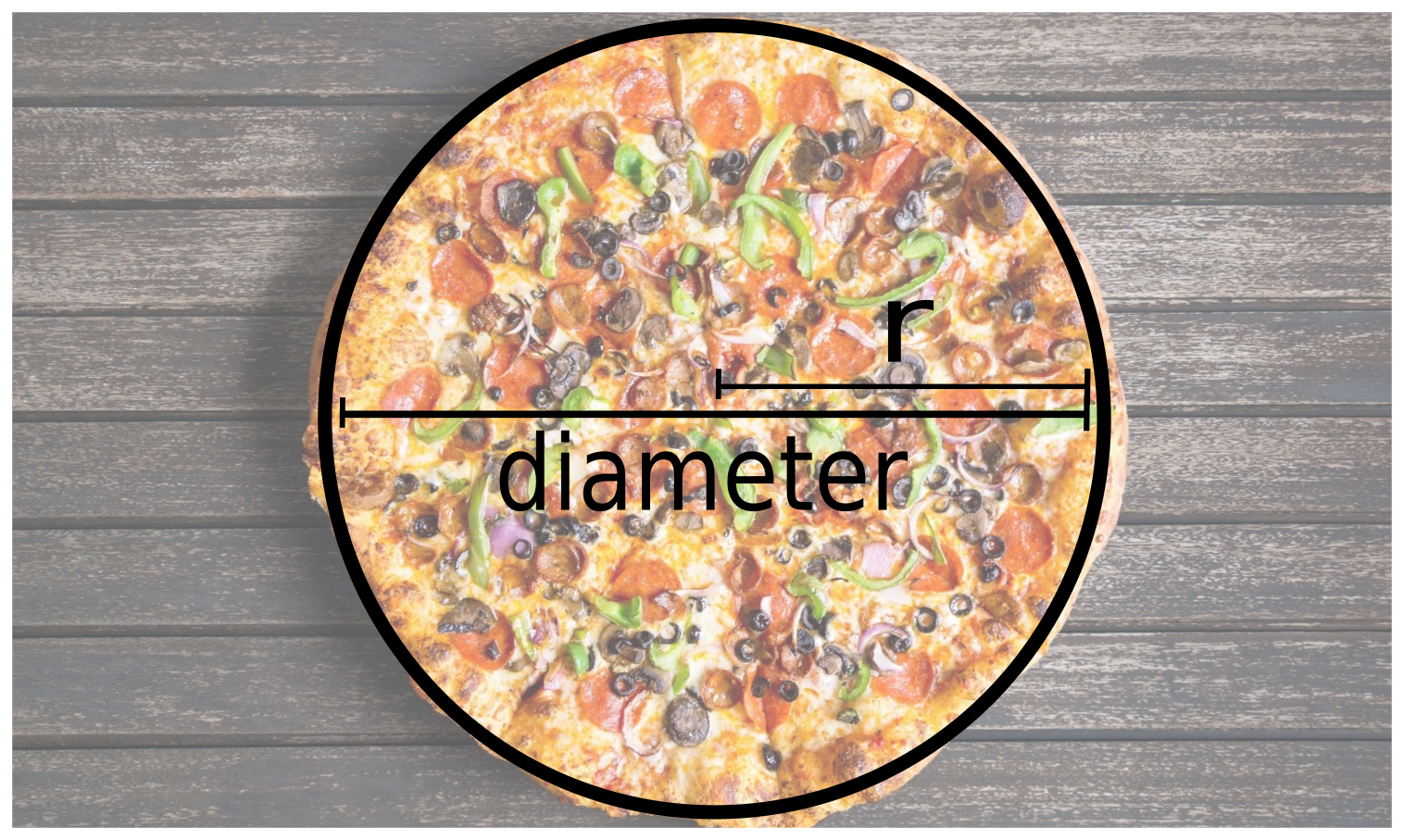# CS21 Lab 1: First programs

## Due Saturday, September 15, before midnight

Make sure all programs are saved to your cs21/labs/01 directory! Files outside that directory will not be graded.

$update21$ cd ~/cs21/labs/01/
$pwd /home/username/cs21/labs/01$ ls
ops.py
pizza.py
QUESTIONS-01.txt

### Programming Tips

As you write your first programs, start using good programming practices now:

• Use a comment at the top of the file to describe the purpose of the program.
• Use variable names that describe the contents of the variables.
• Write your programs incrementally and test them as you go. This is really crucial to success: test, test again, and then test some more!
• Don't assume that if your program passes the sample tests we provide that it is completely correct. Come up with your own test cases and verify that the program is producing the right output on them.

You are in charge of writing a script for the daily specials at a new restaurant in Swarthmore: Corner Cafe. In menu.py, write a program that will ask the restaurant for three pieces of information: the soup of the day, the special of the day, and the dessert of the day. Your program should then use this information to generate a short description of the daily specials (i.e. a restaurant madlib).

Here are two examples (user input in bold):

$python3 menu.py Soup: wild rice Special: salmon Dessert: peach cobbler Welcome to Corner Cafe! Our soup of the day is wild rice The special tonight is salmon For dessert, try our world-famous peach cobbler$ python3 menu.py
Soup: cup noodles
Special: pizza
Dessert: M&Ms

Welcome to Corner Cafe!
Our soup of the day is cup noodles
The special tonight is pizza
For dessert, try our world-famous M&Ms

Note: you can use print() with no arguments to print a blank line.

### 2. Python Math Operators

Just to get comfortable with some python3 math operators, write a program called ops.py that asks the user for two integer factors, and shows the results of applying / (division), ** (exponentiation), and % (mod) to the factors.

Here's a few quick examples (user input in bold):

$python3 ops.py Please enter two integers. Factor #1: 4 Factor #2: 10 4 / 10 = 0.4 4 ** 10 = 1048576 4 % 10 = 4$ python3 ops.py

Factor #1: 4
Factor #2: 3

4 / 3 = 1.3333333333333333
4 ** 3 = 64
4 % 3 = 1

$python3 ops.py Please enter two integers. Factor #1: 17 Factor #2: 3 17 / 3 = 5.666666666666667 17 ** 3 = 4913 17 % 3 = 2 Tip: explore these three operators in the interpreter (i.e. just run python3 in the terminal) before writing your code. ### 3. Group Pizza In pizza.py, write a short program that helps friends divide the bill after ordering pizza. Your program should ask the user for the number of friends (excluding the user), the number of pizzas, and the tip (as a percentage). Each pizza is $12 and there is an additional $10 fixed delivery fee. Your program should output three calculations: the total cost (including tip on both the pizzas and the delivery fee), the cost per person, and the average number of slices per person, assuming 8 slices per pizza. Here are two examples (user input in bold): $ python3 pizza.py
Number of friends: 15
Number of pizzas: 5
Tip (%): 18
--------------------------------
Total: $82.6 Cost per Person:$ 5.1625
Slices per Person: 2.5

$python3 pizza.py Number of friends: 6 Number of pizzas: 42 Tip (%): 15 -------------------------------- Total:$ 591.0999999999999
Cost per Person: $84.44285714285714 Slices per Person: 48.0 You do not have to worry about having an even number of pennies (i.e., exactly two decimal places). We will learn how to do that later in the semester. You should assume that the number of people and the number of pizzas will be whole numbers (integers), but the tip is real valued. ### 4. Pizza Area Building on the previous problem, you are tasked with computing the area of each pizza (in square inches). Modify your pizza.py program to include an additional question for the user: the diameter (in inches) of the pizzas (this could be a real number). Then use this value to print out the area of one pizza. The formula for the area of a circle of radius r is: area of a circle = π ⋅ r2 Here is a diagram that shows the diameter and radius of a pizza:The previous examples are modified to show this additional output: $ python3 pizza.py
Number of friends: 15
Number of pizzas: 5
Tip (%): 18
Pizza diameter (inches): 14
--------------------------------
Total: $82.6 Cost per Person:$ 5.1625
Slices per Person: 2.5
Area of one pizza: 153.93804002589985

$python3 pizza.py Number of friends: 6 Number of pizzas: 42 Tip (%): 15 Pizza diameter (inches): 77 -------------------------------- Total:$ 591.0999999999999
Cost per Person: \$ 84.44285714285714
Slices per Person: 48.0
Area of one pizza: 4656.625710783471

Note: to use pi correctly in your program, you will need to import the math library. If you haven't seen this in class yet, you just need to add import math at the top of your program. You can insert this after the comment at the start of the program but it should be before you define your main function.

Here's an interactive example showing some variables and functions from the math library:

>>> import math
>>> math.sqrt(4)
2.0
>>> math.sqrt(64)
8.0
>>> math.sqrt(37)
6.082762530298219
>>> math.pi
3.141592653589793
>>> math.e
2.718281828459045

Each lab has a short questionnaire at the end. Please edit the QUESTIONS-01.txt file in your cs21/labs/01 directory and answer the questions in that file.
Don't forget to run handin21 to turn in your lab files! You may run handin21 as many times as you want. Each time it will turn in any new work. We recommend running handin21 after you complete each program or after you complete significant work on any one program.